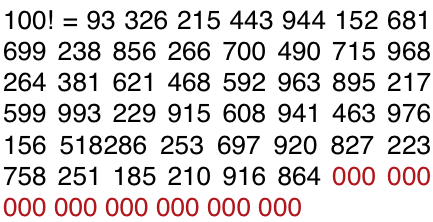Legendre’s Formula

There is a theorem in number theory known as Legendre’s Formula. It states that if N is a positive integer and p is a prime number, then the highest power of p that divides N! is given by the following formula

$e_{p} = \sum_{i = 1}^{\infty}\lfloor{\frac{N}{p^{i}}}\rfloor$

Translating math to english this reads “The highest power of p that divides N! is the infinite sum of the floor of N divided by p to the current exponent”

We know that the infinite sum will eventually reach a terminal value of 0 as at some point, we will reach a large enough exponent for p that the result of dividing n by p raised to i is less than 1. And taking the floor of any value less than 1 will give us 0. Therefore, we do not need to worry about adding up an infinite amount of quotients unless our N is astronomically large.

This formula will allow us to determine the highest power of p in N!. But what about non-prime values? We can take the prime factorization of our target number and run separate instances of the function. Since 10 is the product of 2 and 5, we can simply take the minimum value returned by running the formula on 2, and then 5.

$\min(\sum_{i = 1}^{\infty}\lfloor{\frac{N}{2^{i}}}\rfloor,\sum_{i = 1}^{\infty}\lfloor{\frac{N}{5^{i}}}\rfloor)$

But, knowing that powers of 5 will always be larger than powers of two, the number returned by Legendre’s formula for 5 will always be smaller. Therefore, we can dispense with the minimum function altogether and simply find out how many exponents of 5 divide into the factorial. This will give us the number of trailing zeros.

Example Problems

Trailing zeros in 100!

$\sum_{i = 1}^{\infty}\lfloor{\frac{100}{5^{i}}}\rfloor) = \lfloor\frac{100}{5}\rfloor + \lfloor\frac{100}{5^{2}}\rfloor = 20 +4 = 24$

We have 24 trailing zeros in 100!

Trailing zeros in 95!

$\sum_{i = 1}^{\infty}\lfloor{\frac{95}{5^{i}}}\rfloor) = \lfloor\frac{95}{5}\rfloor + \lfloor\frac{95}{5^{2}}\rfloor = 19 + 3 = 22$

We have 22 traling zeros in 95!

Trailing Zeros Coding Question

Suppose we want to write a program to find the number of trailing zeros in n!. We can simply translate our algorithm into code. We will use a while loop to iterate until the floor of our number divided by 5 to our current exponent yields zero. While iterating we will track the sum and return it upon termination. Leetcode features this exact question.

def trailingZeroes(self, n: int) -> int:
power = 1
sum = 0
while (n//(5**power) != 0):
sum+=(n//(5**power))
power+=1
return sum